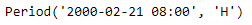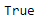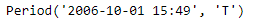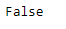Open in App
Not now

# Python | Pandas Period.is_leap_year

• Last Updated : 06 Jan, 2019

Python is a great language for doing data analysis, primarily because of the fantastic ecosystem of data-centric python packages. Pandas is one of those packages and makes importing and analyzing data much easier.

Pandas` Period.is_leap_year` attribute checks for the given Period object, if the year in the object is a leap year or not. It return `True` if the following year is a leap year else it return `False`

Syntax : Period.is_leap_year

Parameters : None

Return : boolean

Example #1: Use `Period.is_leap_year `attribute to check if the date present in the given Period object is a leap year or not.

 `# importing pandas as pd``import` `pandas as pd`` ` `# Create the Period object``prd ``=` `pd.Period(freq ``=``'H'``, year ``=` `2000``, month ``=` `2``, day ``=` `21``, hour ``=` `8``)`` ` `# Print the Period object``print``(prd)`

Output :Now we will use the `Period.is_leap_year` attribute to check if the date present in the prd object is a leap year or not.

 `# check for leap year``prd.is_leap_year`

Output :As we can see in the output, the `Period.is_leap_year `attribute has returned `True` indicating that the following year in the prd object is a leap year.

Example #2: Use `Period.is_leap_year` attribute to check if the date present in the given Period object is a leap year or not.

 `# importing pandas as pd``import` `pandas as pd`` ` `# Create the Period object``prd ``=` `pd.Period(freq ``=``'T'``, year ``=` `2006``, month ``=` `10``, hour ``=` `15``, minute ``=` `49``)`` ` `# Print the Period object``print``(prd)`

Output :Now we will use the `Period.is_leap_year` attribute to check if the date present in the prd object is a leap year or not.

 `# check for leap year``prd.is_leap_year`

Output :As we can see in the output, the `Period.is_leap_year `attribute has returned `False` indicating that the following year in the prd object is not a leap year.

My Personal Notes arrow_drop_up